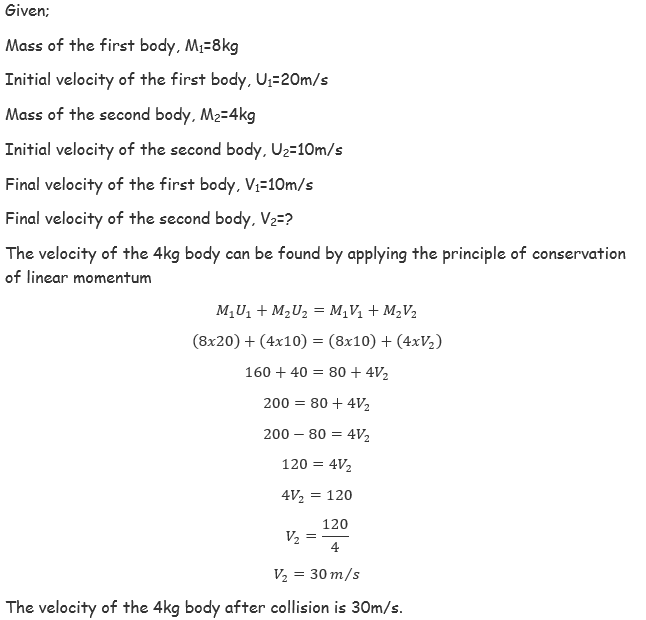error: Content is protected !!

# PHYSICS: FORM TWO: Topic 7 - NEWTON'S LAW OF MOTION

TOPIC 7: NEWTON'S LAW OF MOTION

The Concept of Inertia
Explain the concept of inertia
Inertia is the tendency of a body at rest to resist motion when forced to move and a body in motion to continue moving when abruptly stopped.A good example is a passenger sitting on a seat in a bus at rest. When the bus starts moving the passenger will experience a backward push. This is because the body of the passenger was continuing in its state of rest when the bus was starting its motion. Similarly, when the bus stops abruptly the passenger will experience a forward push because as the bus stops, the passenger’s body was still in state of motion. The above situation is described as inertia.
Verification of Newton's First Law of Motion
Verify Newton's first law of Motion
Newton's first law can be verified by performing the below simple experiment.
Activity 1
Experiment
Aim: To verify Newton’s 1st law of motion.
Materials and apparatus: Glass, manila card and small coin.
Procedures.
A small coin is placed on a manila card and the card is positioned on top of the glass such that the coin is directly positioned over the open mouth of the bottle.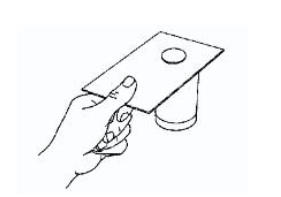Flick the card at C. Make sure that the card is not tilted by moving the finger in the horizontal plane.
Observation: When the card is flicked away quickly by finger, the coin drops neatly into the glass. The coin dropped into the glass because there was no force applied on it when the card was flipped.
Conclusion: The coin continued to be at rest as the card was flicked quickly. This experiment verify Newton’s 1st law of motion.
The Concept of Linear Momentum
Explain concept of linear momentum
Linear momentum is one of important aspect used to explain the motion of a body in a straight line. Linear momentum is obtained as the product of mass and velocity A body with a larger mass and the one with smaller mass will possess different amount of momentum when moving with same velocity. A body with smaller mass can be stopped easily compared to the one with the larger mass. That is to say, larger mass bodies possess large momentum than smaller mass bodies. Linear momentum has both magnitude and direction. The SI unit of linear momentum is simply the combination of the individual SI units of mass and velocity. Therefore, the SI unit of linear momentum is Kilogram -Metre per second (kgm/s). The linear momentum is determined by measuring the mass of the body and its velocity. A body with large mass and moving with high velocity possess large momentum. Since them mass of the body remains constant in most of the times, the momentum will only change if its velocity changes. What is a linear momentum of a 10ton lorry moving with 60m/s? A ball weighing 3kg has been kicked and found to move at 35m/s. Calculate the linear momentum of the ball. This law explains the effect of the external force on the momentum of the body. The applied force can increase or decrease the momentum of the body. The rate of the momentum change depends on the amount of the applied force.
Verification of Newton's Second Law of Motion
Verify Newton's second law of Motion
Newton's second law can be verified by observing the changes between the applied force and acceleration. This can be verified by through the trolley experiment.
A trolley experiences an acceleration when an external force is applied to it. The aim of this data logging experiment is to explore the relationship between the magnitudes of the external force and the resulting acceleration.
Apparatus and materials
• Light gate, interface and computer
• Dynamics trolley
• Pulley and string
• Slotted masses, 400 g
• Mass, 1 g
• Clamp
• Ruler
• Double segment black card (see diagram)
Take care when masses fall to the floor. Use a box or tray lined with bubble wrap (or similar) under heavy objects being lifted. This will prevent toes or fingers from being in the danger zone.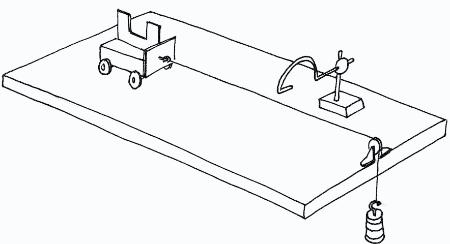Procedure
1. Select the falling mass to be 100 g. Pull the trolley back so that the mass is raised to just below the pulley. Position the light gate so that it will detect the motion of the trolley soon after it has started moving.Set the software to record data, then release the trolley. Observe the measurement for the acceleration of the trolley.
2. Repeat this measurement from the same starting position for the trolley several times. Enter from the keyboard '1'( 1 newton) in the force column of thetable.
3. Transfer 100 g from the trolley to the slotted mass, to increase it to 200 g. Release the trolley from the same starting point as before. Repeat this several times. Enter '2' (2 newtons) in the force column of the table.
4. Repeat the above procedure for slotted masses of 300 g and 400 g.

Difference between Elastic and Inelastic Collisions
Distinguish between Elastic and Inelastic Collisions
Elastic collision
This is the type of collision whereby each body moves with a separate velocity after collision. In this type of collision both energy and momentum are conserved.Inelastic collision
Is the type of collision whereby all bodies move with the same velocity after collision. This velocity is known as common velocity. In this type of collision energy is not conserved, only momentum is conserved.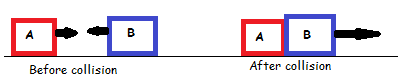Impulse
Impulse is the change of momentum which is given as the product of force and the time taken to change momentum.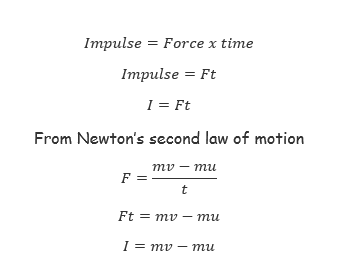Therefore, impulse is the change in momentum of a body which is acted by a force. The forces which act over short time intervals are called impulsive forces.

The Principle of Conservation of Linear Momentum
State the principle of conservation of linear Momentum
Principle of conservation of linear momentum states that, “When two or more bodies acts upon one another without external forces acting on them, total momentum before collision is equal to total momentum after collision”
Consider bodies A and B of mass Ma and Mb and initial velocities Ua and Ub respectively in motion before colision;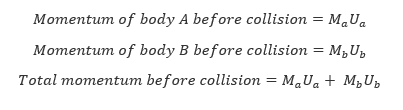After collision, the two bodies moved with velocities Va and Vb in opposite direction to each other as shown below;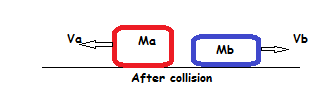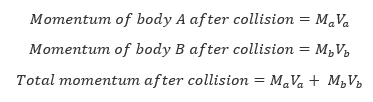During collision, there are large changes of forces within a very short time (impulses).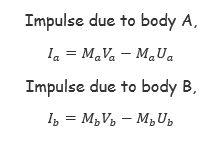Since there are no external forces acting on the system;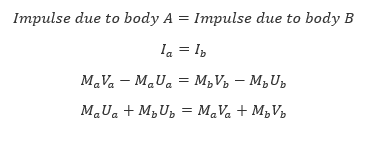The Principle of Conservation of Linear Momentum in Solving Problems
Apply the principle of conservation of linear momentum in solving problems
The principle of conservation of linear momentum can be used in solving various mathematical challenges.
Example 1
A body of mass 8kg moving with a velocity of 20m/s collides with another body of mass 4kg moving with a velocity of 10m/s in a head-on collision. If after collision the 8kg body moved with 10m/s, what is the velocity of the 4kg body after collision?